# hyperbolic cosine

## exponential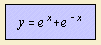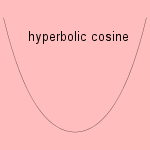Usually one adds a factor 1/2 to the formula, to form the hyperbolic cosine cosh(x) (or ch(x)). This is the construction of the mid-points of the vertical line segments between ex and e-x.
In a Whewell equation the curve can be written as s = tan f.

The curve is generally known as the catenary1). Other names that have been given to the curve are chainette (French) and funicular curve.
The curve is the result of a (perfect, uniform) flexible and inextensible chain between two supports, as result of gravity. Galileo was wrong supposing this curve is the parabola. It was Jungius (1669) who did prove this.
In 1690 Jakob Bernoulli challenged his colleagues to find this 'chain-curve'. Christiaan Huygens as well as Johann Bernoulli and Leibniz succeeded independently to describe the phenomenon mathematically (1691).
It was Huygens who used the word 'catenary'2) for the first time, in a letter to Leibniz (1690).
There was also a man named David Gregory who wrote a treatise on the curve (1690).

There are several ways to show that the chain has the form of the hyperbolic cosine. One way is to look at the vertical and horizontal component of the forces in a small piece of the chain. Solving the resulting differential equation3) gives the catenary. The form is independent of the length and other qualities of the chain.
An alternate way is to calculate the minimal potential energy, or - which is equivalent - have the center of gravity as low as possible. By way of calculus of variations the minimum of the found integral can be derived 4), and the catenary results.

A catenary, upside down, forms a state with maximal potential energy. A bridge, constructed as a catenary with exactly sawn wooden blocks, moves in the same manner as the chain, without breaking down. This construction is of course much less stable than the hanging chain.

When a sail is attached on two horizontal bars, and the wind blows in a direction perpendicular to the bars, a catenary formed sail occurs. For this reason Jakob Bernoulli named the curve the vťlaire.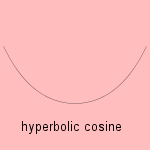In 1819 a building was erected in 1819, with the form of a catenary. The building is called the Rotunda, and was designed by John Nash as a specialized museum for military models. Its form seems to be influenced by military bell tents.

Some relationships with other curves are the following:

The catenary can be generalized to the alysoid.

The revolution of the curve is the catenoid.

notes

1) In Italian: catenaria

2) From catenaria (Lat.).

3) Differential equation: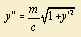with m the mass density and c the stress in the horizontal direction in the chain. Blij 1975 p. 301.

4) Minimizing differential equation: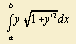with boundary conditions y(a) = y(b) leads to equation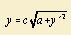Arthurs 1975 p.14.